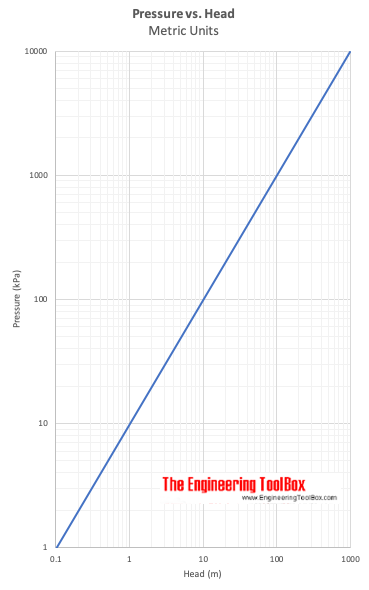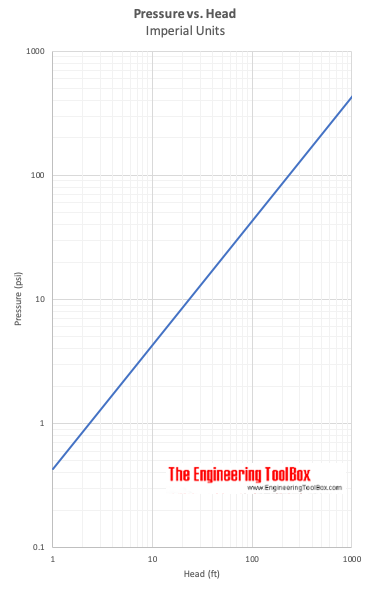Engineering ToolBox - Resources, Tools and Basic Information for Engineering and Design of Technical Applications!

## Static pressure vs. pressure head in fluids.

Pressure indicates the normal force per unit area at a given point acting on a given plane. Since there is no shearing stresses present in a fluid at rest - the pressure in a fluid is independent of direction.

For fluids - liquids or gases - at rest the pressure gradient in the vertical direction depends only on the specific weight of the fluid.

How pressure changes with elevation in a fluid can be expressed as

Δp = - γ Δh                                            (1)

where

Δp = change in pressure (Pa, psi)

Δh = change in height (m, in)

γ = specific weight of fluid (N/m3, lb/ft3)

The pressure gradient in vertical direction is negative - the pressure decrease upwards.

### Specific Weight

Specific Weight of a fluid can be expressed as:

γ = ρ g                                             (2)

where

ρ = density of fluid (kg/m3, slugs/ft3)

g = acceleration of gravity (9.81 m/s2, 32.174 ft/s2)

In general the specific weight - γ - is constant for fluids. For gases the specific weight - γ - varies with elevation (and compression).

The pressure exerted by a static fluid depends only upon

• the depth of the fluid
• the density of the fluid
• the acceleration of gravity

### Static Pressure in a Fluid

For a incompressible fluid - as a liquid - the pressure difference between two elevations can be expressed as:

Δp = p2 - p1

= - γ (h2 - h1)                                     (3)

where

p2 = pressure at level 2  (Pa, psi)

p1 = pressure at level 1   (Pa, psi)

h2 = level 2    (m, ft)

h1 = level 1   (m, ft)

(3) can be transformed to:

Δp = p1 - p2

= γ (h2 - h1)                                      (4)

or

p1 - p2 = γ Δh                                     (5)

where

Δh = h2 - h1 = difference in elevation - the dept down from location h2 to h1  (m, ft)

or

p1 = γ Δh + p2                                           (6)

#### Example - Pressure in a Fluid

The absolute pressure at water depth of 10 m can be calculated as:

p1 = γ Δh + p2

= (1000 kg/m3) (9.81 m/s2) (10 m) + (101.3 kPa)

= (98100 kg/ms2 or Pa) + (101300 Pa)

= 199400 Pa

= 199.4 kPa

where

ρ = 1000 kg/m3

g = 9.81 m/s2

p2 = pressure at surface level = atmospheric pressure = 101.3 kPa

The gauge pressure can be calculated by setting p2 = 0

p1 = γ Δh + p2

= (1000 kg/m3) (9.81 m/s2) (10 m)

= 98100 Pa

= 98.1 kPa

(6) can be transformed to:

Δh = (p2 - p1) / γ                                                (7)

Δh express head - the height difference  of a column of fluid of specific weight - γ - required to give a pressure difference Δp = p2 - p1.

#### Example - Pressure vs. Head

A pressure difference of 5 psi (lbf/in2) is equivalent to head in water

(5 lbf/in2) (12 in/ft) (12 in/ft) / (62.4 lb/ft3)

= 11.6  ft of water

(5 lbf/in2) (12 in/ft) (12 in/ft) / (847 lb/ft3)

= 0.85  ft of mercury

Specific weight of water is 62.4 (lb/ft3) and specific weight of mercury is 847 (lb/ft3).## Related Topics

• ### Fluid Mechanics

The study of fluids - liquids and gases. Involving velocity, pressure, density and temperature as functions of space and time.
• ### Pumps

Piping systems and pumps - centrifugal pumps, displacement pumps - cavitation, viscosity, head and pressure, power consumption and more.

## Related Documents

• ### Darcy-Weisbach Equation - Major Pressure and Head Loss due to Friction

The Darcy-Weisbach equation can be used to calculate the major pressure and head loss due to friction in ducts, pipes or tubes.
• ### Efficiency in Pumps or Fans

The overall pump and fan efficiency is the ratio power gained by the fluid to the shaft power supplied.
• ### Hydropower

Power potential vs. head and flow rate.
• ### Hydrostatic Pressure vs. Depth

Depth and hydrostatic pressure.
• ### PE, PEH and PVC Pipes - Pressure Loss vs. Water Flow Diagram

Pressure loss (bar/100 m) and velocy in PE, PEH or PVC pipes with water flow.
• ### Potential Energy - Hydropower

Elevation and potential energy in hydropower.

Static pressure graphical presentation throughout a fluid flow system.
• ### Pressure to Head Unit Converter

Pressure vs. head units - like lb/in2, atm, inches mercury, bars, Pa and more.
• ### Pumps - Head vs. Pressure

Converting head (ft or m) to pressure (psi or bar, kg/cm2) and vice versa.
• ### Pumps - NPSH (Net Positive Suction Head)

An introduction to pumps and the Net Positive Suction Head (NPSH).

• ### Pumps - Suction Head vs. Altitude

The suction head of a water pump is affected by its operating altitude.
• ### Pumps and Fans - Energy Equation and Head Rise

The energy equation can be used to calculate the head rise in pumps and fans.
• ### System Curve and Pump Performance Curve

Utilize the system curve and the pump performance curve to select the proper pump for a particular application.
• ### Types of Fans - Capacity Ranges

Centrifugal, axial and propeller fans and their capacity ranges.

• ### Water Pressure vs. Head

Pressure in pounds per square inch (psi) vs. head in feet of water (fth2o).

## Engineering ToolBox - SketchUp Extension - Online 3D modeling!

Add standard and customized parametric components - like flange beams, lumbers, piping, stairs and more - to your Sketchup model with the Engineering ToolBox - SketchUp Extension - enabled for use with older versions of the amazing SketchUp Make and the newer "up to date" SketchUp Pro . Add the Engineering ToolBox extension to your SketchUp Make/Pro from the Extension Warehouse !

We don't collect information from our users. More about

## Citation

• The Engineering ToolBox (2003). Static Pressure vs. Head. [online] Available at: https://www.engineeringtoolbox.com/static-pressure-head-d_610.html [Accessed Day Month Year].

Modify the access date according your visit.

9.27.11